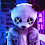Maths Std 10 Chapter 03 - Maths Science Corner

# Maths Science Corner

Math and Science for all competitive exams

# Textbook Chapter

On maths science corner you can now download new NCERT 2019 Gujarati Medium Textbook Standard 10 (Class 10) Maths Chapter 03 Dwichal Surekh Samikaran (Pair of Linear Equation in Two Variables) Textbook Chapter in pdf form for your easy reference.

On Maths Science Corner you will get all the printable study material of Maths and Science Including answers of prayatn karo, Swadhyay, Chapter Notes, Unit tests, Online Quiz etc..

This material is very helpful for preparing Competitive exam like Tet 1, Tet 2, Htat, tat for secondary and Higher secondary, GPSC etc..

Highlight of the chapter

3.1 Introduction

3.2 Pair of Linear Equations in Two variables

3.3 Graphical Method of Solution of a pair of linear equations in two variables

3.4 Algebraic methods of solving a a pair of linear equations in two variables

3.4.1 Substitution method

3.4.2 Elimination method

3.4.3 Cross - multiplication method

3.5 Equations reducible to a pair of linear equations in two variables

3.6 Summary

You will be able to learn above topics in Chapter 03 of Maths Standard 10 (Class 10) Textbook chapter.

Today Maths Science Corner is giving you the textbook Chapter 03 of Maths Standard 10 (Class 10) in pdf format for your easy reference.

Maths Std 10 Chapter 03

You can get Std 6 Material from here.

You can get Std 7 Material from here.

You can get Std 8 Material from here.

You can get Std 10 Material from here.

#### 1 comment:

1.